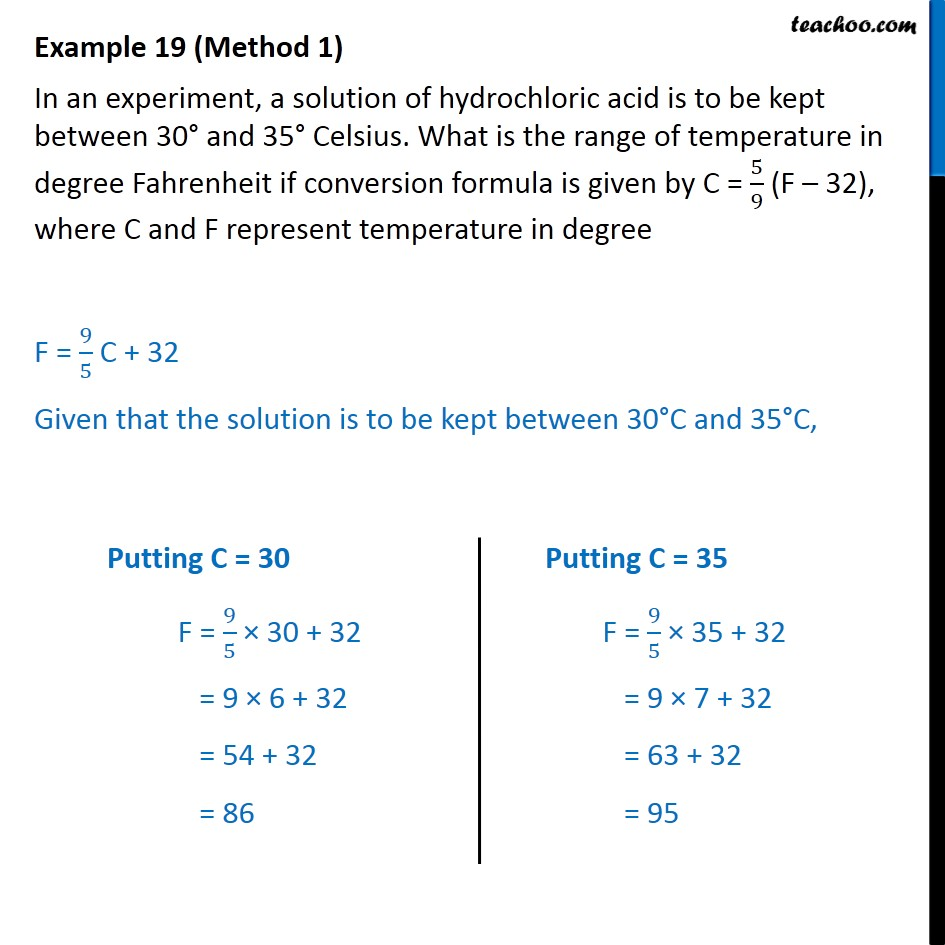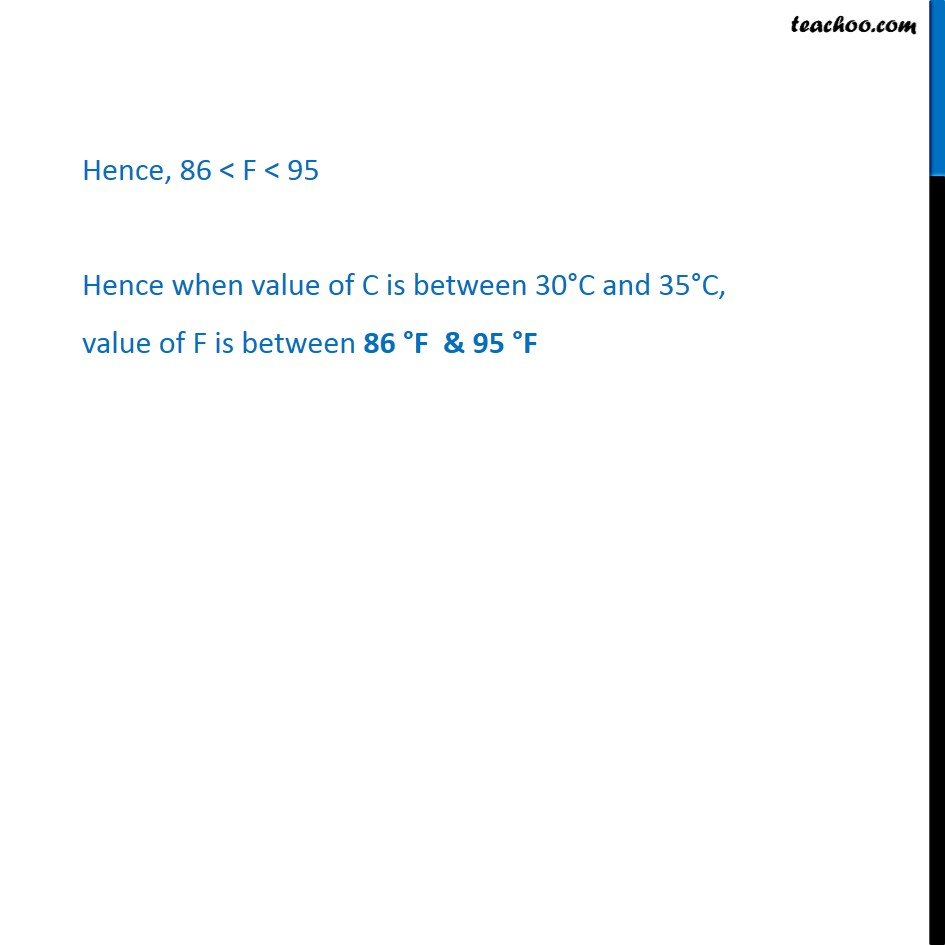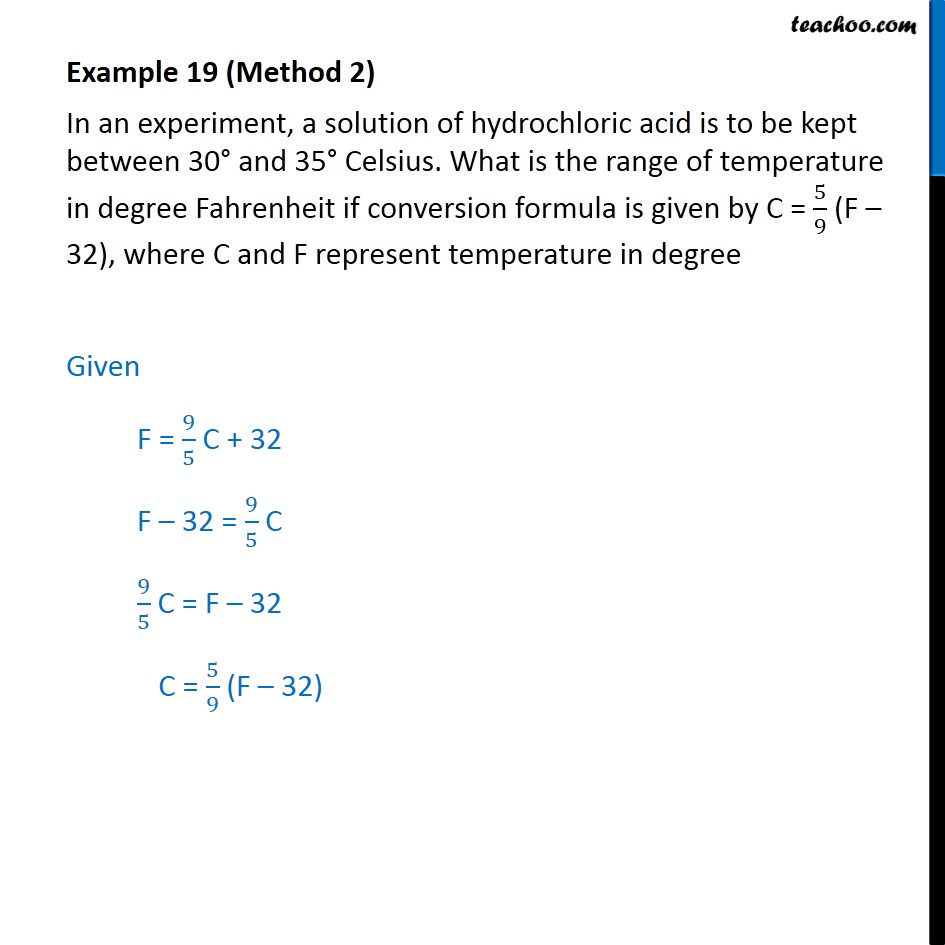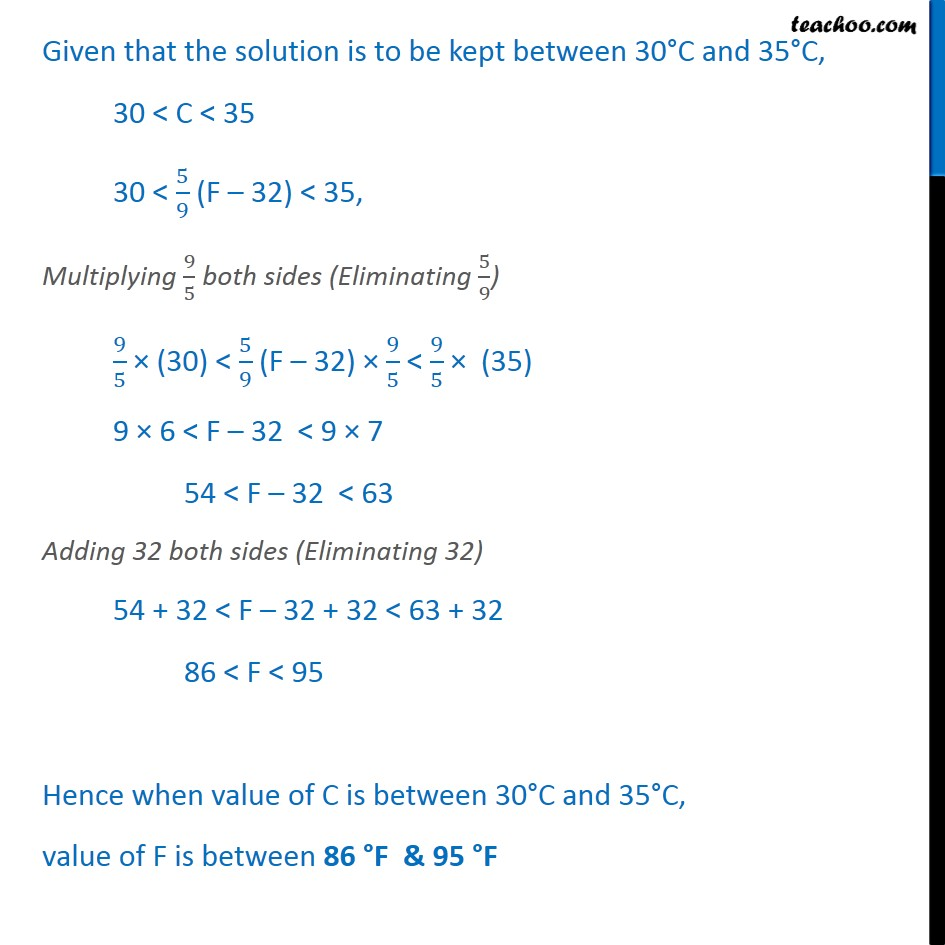Examples

Chapter 5 Class 11 Linear Inequalities
Serial order wiseLearn in your speed, with individual attention - Teachoo Maths 1-on-1 Class

### Transcript

Example 12 (Method 1) In an experiment, a solution of hydrochloric acid is to be kept between 30° and 35° Celsius. What is the range of temperature in degree Fahrenheit if conversion formula is given by C = 5﷮9﷯ (F – 32), where C and F represent temperature in degree F = 9﷮5﷯ C + 32 Given that the solution is to be kept between 30°C and 35°C, Hence, 86 < F < 95 Hence when value of C is between 30°C and 35°C, value of F is between 86 °F & 95 °F Example 12 (Method 2) In an experiment, a solution of hydrochloric acid is to be kept between 30° and 35° Celsius. What is the range of temperature in degree Fahrenheit if conversion formula is given by C = 5﷮9﷯ (F – 32), where C and F represent temperature in degree Given F = 9﷮5﷯ C + 32 F – 32 = 9﷮5﷯ C 9﷮5﷯ C = F – 32 C = 5﷮9﷯ (F – 32) Given that the solution is to be kept between 30°C and 35°C, 30 < C < 35 30 < 5﷮9﷯ (F – 32) < 35, Multiplying 9﷮5﷯ both sides (Eliminating 5﷮9﷯) 9﷮5﷯ × (30) < 5﷮9﷯ (F – 32) × 9﷮5﷯ < 9﷮5﷯ × (35) 9 × 6 < F – 32 < 9 × 7 54 < F – 32 < 63 Adding 32 both sides (Eliminating 32) 54 + 32 < F – 32 + 32 < 63 + 32 86 < F < 95 Hence when value of C is between 30°C and 35°C, value of F is between 86 °F & 95 °F